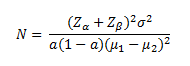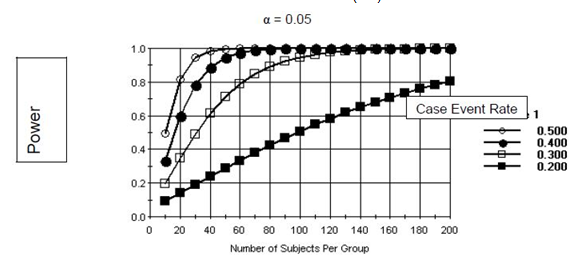# Analysis of Means

Under the assumptions that observations are independent and the variance is equal in two groups, the study size to detect differences between two means can be calculated (8). The study size formula for a two-group comparison is:In the above equation, µ1- µ2  is the expected difference between two means and σ2 is the common variance of the continuous variable.

Power is lowered as the proportion of the population (a) with an attribute becomes further away from 0.5, which in turn indicates that the proposed sample size will only be sufficient to detect larger effects sizes as the prevalence of the attribute decreases. In general, effect sizes that can be detected in this study are small to moderate (if the whole study size is used) and moderate to large (if a subgroup analysis is performed).

Below we present a graph showing the power of a two-group comparison of a continuous measure as a function of the effect size [(µ1- µ2)/σ] and a common sample size in the two groups.

Comparison of Two Group Means

As a Function of Effect Size and the Sample Size

α = 0.05As can be seen from the graph, if substantial effect sizes can be hypothesized, the number of required samples for a study will be small. For instance, if the effect size is one half of a standard deviation, a sample size of at least 80 per group will be sufficient to detect this effect when testing at the a= 0.05 level. To have adequate power to detect smaller effects will require larger sample sizes. It is likely that most effects sizes being proposed in the LTRC will fall into the range presented in the above figure.

## Horizontal Tabs

This page is associated with a larger collection of pages. You can view them as a whole here.